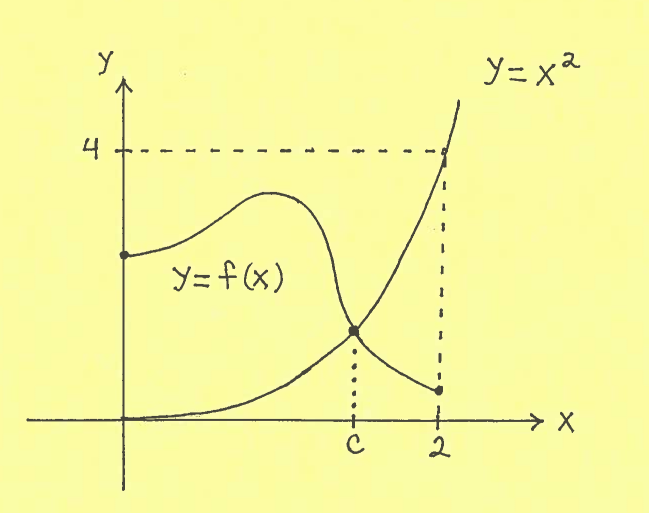SOLUTION 16: Consider the graphs of $y=f(x)$ and $y=x^2$ on the interval $[0, 2]$ with $f(0)>0$ and $f(2)<4$.Let function $$g(x)= f(x)-x^2 \ \ \ \ and \ choose \ \ \ \ m=0$$
Function $g$ is continuous on the interval $[0, 2]$ since it is the DIFFERENCE of continuous functions. Note that $$g(0) = f(0) - (0)^2 = f(0)>0$$ and $$g(2)=f(2) - (2)^2 = f(2)-4 < 0$$
i.e., $m=0$ is between $f(0)$ and $f(2)$.

The assumptions of the Intermediate Value Theorem have now been met on the interval $[0, 2]$, so we can conclude that there is some number $c$ in the interval $[0, 2]$ which satisfies $$g(c)=m$$ i.e., $$f(c)-c^2 =0 \ \ \ \ \longrightarrow \ \ \ \ f(c)=c^2$$ This completes the proof.